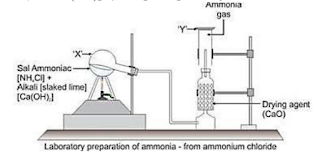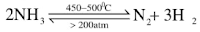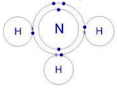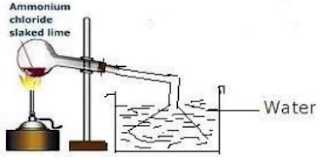# ICSE Solutions for Chapter 9 Study of Compounds: Ammonia Class 10 Selina Chemistry

### Exercise 9 A

Question 1: Draw a labelled diagram and give a balanced equation for the lab. Preparation of ammonia. Also state physical properties of ammonia.

Solution 1: Physical properties of ammonia are:
Color: Colourless
Odour: Strong, Pungent chocking smell
Taste: Slightly bitter (alkaline) taste
Physiological action: Non-Poisonous
Density: V.D = 8.5 Lighter than air
Nature: Alkaline
Liquefaction: easily liquefied at 10°C by compressing it at 6 atm. Pressure
Boiling Point: Liquid ammonia boils at -33.5°C
Freezing Point: Solid NH3 melts at –77.7°C
Solubility: Highly soluble in water, l vol of water dissolves about 702 vols. of ammonia at 20°C and 1 atm. pressure.
Reaction:
2NH4Cl + Ca(OH)2 → CaCl2 + 2H2 + 2NH3Question 2: (a) Is ammonia denser or less dense than air?
(b) What property of ammonia is demonstrated by the fountain experiment? (c) Write the balanced equation for the reaction between ammonia and sulphuric acid.

Solution 2: Ammonia is less dense than air. By Fountain Experiment, we demonstrate the high solubility of ammonia gas in water.
Balanced equation for the reaction between ammonia and sulphuric acid is:
2NH3 + H2SO→ (NH4)2SO4

Question 3: Pick the odd member from the list giving reasons: (a) Ammonia, sulphur dioxide, hydrogen chloride, carbon dioxide. (b) Copper oxide, aluminium oxide, sodium oxide, magnesium oxide.

Solution 3: (a) Ammonia is basic in nature.
(b) Copper oxide, because CuO is less reactive, can be reduced by C, CO or by hydrogen whereas Al2O3, Na2O, MgO are reduced by electrolysis.

Question 4: The following reactions are carried out:
A: Nitrogen + metal compound X.
B: X + water → ammonia + another compound
C: Ammonia + metal oxide → metal + water + N2
One metal that can be used for reaction A is magnesium.
(a) write the formula of the compound X formed
(b) write the correct balanced equation for reaction B where X is the compound formed.
(c) what property of ammonia is demonstrated by reaction C?

Solution 4: (a) The formula of the compound is Mg; N2
(b) Balanced equation : Mg3N2 + 6 H2O → 3 Mg(OH)2 + 2NH3
(C) Ammonia is a reducing agent and reduces less active metal oxide to its respective metal.

Question 5: Ammonium salts decompose on heating. What other property do ammonium salts have in common?

Solution 5: Reducing property.

Question 6: State what you observe when a piece of moist red litmus paper is placed in a gas jar of ammonia.

Solution 6: When a piece of moist red litmus paper is placed in a gas jar of ammonia it turns blue.

Question 7: A gas 'P' gives dense white fumes with chlorine. Its aqueous solution gives a blue colour with copper (II) hydroxide (a) Name the gas P. (b) Give its formula (c) Give three uses of P.

Solution 7: (a) The gas is ammonia.
(b) The formula is NH3.
(c) Uses of ammonia:
It is used in the industrial preparation of nitric acid by Ostwald process.
It is used in the manufacture of fertilizers such as ammonium sulphate, ammonium nitrate, ammonium phosphate.
It is used in the manufacture of sodium carbonate by the Solvay process.
NaCl + NH+ CO2 + H2O → NaHCO3 + NH4Cl

Question 8: Ammonia solution in water gives a blue precipitate when it combines with a solution of copper salt. The blue precipitate further dissolves in excess of ammonia solution to give an azure blue solution. Explain with the equation.

Solution 8: Equation:
CuSO4 + 2NH4OH → Cu(OH)2⬇️  + [NH4]2SO4
Colour of CuSO4 is pale blue.
Ammonia solution in water gives a blue precipitate when it combines with a solution of copper salt.
The pale blue precipitate of copper hydroxide dissolves in excess of ammonium hydroxide forming tetraamine copper[II] sulphate, an azure blue (deep blue) soluble complex salt.
Cu(OH)2 + (NH4)2SO4 + 2NH4OH →  [Cu(NH3)4]SO4 + 4H2O

Question 9: How do you prove that NH3 contains nitrogen and hydrogen?

Solution 9: Ammonia dissociates into nitrogen and hydrogen at high temperature or by electric sparks.Question 10: Give reasons for the following:
(a) Liquid ammonia is used as a refrigerant in ice plants.
(b) Aqueous solution of ammonia is used for removing grease stains from woollen clothes
(c) Aqueous solution of ammonia gives a pungent smell
(d) Aqueous solution of ammonia conducts electricity

Solution 10: (a) Liquid ammonia takes a lot of energy to vaporize. This heat is taken from the surrounding bodies which are consequently cooled down. Thus it is used as a refrigerant in ice plant.
(b) Ammonia emulsifies or dissolves fats, grease so it is used to remove grease from woollen
clothes.
(c) Aqueous solution of ammonia gives pungent smell because of the presence of ammonia.
(d) Aqueous ammonia when dissolved in water breaks into ions which help in the conductance
of electricity.
NH3 + H2O + NH4OH  ⇌  NH4+ + OH-

Question 11: Copy and complete the following equations.
(a) AIN + H2O →
(b) 2NH3 + 3PbO →
(C) NH3 + 3Cl2 →
(d) NH3 + CO2 →
(i) which property of ammonia is illustrated by equation (c)?
(ii) what important fertilizer is prepared from equation (d) ?

Solution 11: (a) AlN + 3H2O + Al(OH)3 + NH3
(b) 2NH3 + 3PbO → 3Pb + 3H2O + N2
(c) 8NH3 + 3Cl2 → N2 + 6NH4Cl
(d) 2NH3 + CO2 → NH2CONH2 + H2O
(i) Ammonia act as reducing agent is explained by equation (c).
(ii) Urea the nitrogenous fertilizer is prepared from equation (d).

Question 12: Correct the following:
A reddish-brown precipitate is obtained when ammonium hydroxide is added to ferrous sulphate.
(b) Liquid ammonia is a solution of NH3
(c) Finely divided platinum is used in Haber process
(d) Conc. H2SO4 is a drying agent for NH3
(e) Ammonium salts, on heating, decompose to give ammonia.

Solution 12: (a) A Dirty green precipitate of Fe(OH)2 is obtained when ammonium hydroxide is added to ferrous sulphate.
(b) Liquid ammonia is liquefied ammonia.
(c) Finely divided Iron is used in Haber process.
(d) Quicklime is a drying agent for NH3.
(e) Ammonium salts when heated with caustic alkali.

Question 13: What do you observe when ammonium hydroxide is added to the aqueous solution of:
(a) FeSO4 (b) Iron (III) chloride, (c) Lead nitrate (d) Zinc nitrate?

Solution 13: (a) Dirty green ppt. of Ferrous hydroxide is formed which is insoluble in excess of NH4OH.
FeSO4 + 2NH4OH → [NH4]2SO4 + Fe(OH)2

(b) Reddish-brown ppt. of ferric hydroxide is formed which is insoluble in ammonium
hydroxide.
FeCl3 + 3NH4OH → 3NH4Cl + Fe(OH)3

(c) White ppt. of lead hydroxide is formed which is insoluble in NH4OH.
Pb(NO3)2 + 2NH4OH → 2NH4NO3 + Pb(OH)2

(d) White gelatinous ppt. of Zinc hydroxide is formed which is soluble in NH4OH.
Zn(NO3)2 + 2NH4OH → 2NH4NO3 + Zn(OH)2

Question 14: Why is ammonium hydroxide used in qualitative analysis? Give two equations to justify your answer

Solution 14: When the correct amount of ammonium hydroxide is added dropwise to solutions of the metallic salts, ppts. (coloured generally) are formed. They help us to identify their metal ions.
Two equations:
FeSO4 + 2NH4OH → (NH4).2SO4 + Fe(OH)2
(Green) (Dirty green)
shows the presence of Fe2+ ion.
FeCl3 + 3NH4OH → 3NH4Cl + Fe(OH)3
(Brown) (Reddish brown)
shows the presence of Fe+3 ion.

Question 15: Give a chemical test to distinguish between the following:
(a) Ammonium chloride and sodium chloride
(b) Ferric salt and ferrous salt
(c) Sodium sulphate and ammonium sulphate

Solution 15: (a)NH4Cl on strong heating sublimes to form dense white fumes which condense to white
powdery mass on cooler parts of the tube whereas no white fumes on heating NaCl.

(b) When ammonium hydroxide is added dropwise to the solution to be tested.
Ferrous salt gives dirty green ppt.
Ferric salt gives reddish brown ppt of their hydroxides.

(c) (NH4)2SO4 on warming with NaOH sol. gives NH3 gas. Sodium sulphate does not liberate NH3 gas.

Question 16: Give balanced equations for the following conversation:
(a) Ammonia to nitrogen using an acidic gas,
(b) Ammonia to brown gas,
(c) Ammonia to nitrogen trichloride
(d) Ammonia solution to an amphoteric hydroxide
(e) A nitride of a trivalent metal to ammonia

Solution 16: Balanced equations :
(a) 8NH3 + 3Cl2 → N2 + 6NH4Cl(b) 2NO + O2 → 2NO2
Brown gas: NO2

(c) NH3 + 3Cl2 + 3HCl + NCl3
(d) AlCl3 + 3NH4OH → 3NH4Cl + Al(OH)3
(e) AIN + 3H2O →Al(OH)3 + NH3
(f) 3PbO + 2NH3 + 3Pb + 3H2O + N2

Question 17: (a) explain catalytic oxidation of ammonia
(b) Give two reactions to show reducing property of ammonia.

Solution 17: (a) In the presence of Platinum at 800°C, ammonia reacts with oxygen to give nitric oxide and water vapour.
Procedure:
Pass dry ammonia gas and oxygen gas through inlets overheated platinum placed in the combustion tube, which in the heated state emits a reddish glow.
Reaction:2NO + O2 → 2NO2
Observation: Reddish-brown vapours of nitrogen dioxide are seen in the flask due to the oxidation of nitric oxide.
The platinum continues to glow even after the heating is discontinued since the catalytic oxidation of ammonia is exothermic.

(b) Two reactions to show the reducing property of ammonia are:
8NH3 + 3Cl2 → N2 + 6NH4Cl
2NH3 + 3CuO → 3Cu + 3H2O + N2

Question 18: Choose the correct word or phrase from the brackets to complete the following sentences and write balanced equations for the same.
(i) ammonium chloride is a soluble salt prepared by ......................... (precipitation, neutralization]
(ii) when ammonium chloride is heated, it undergoes ...................... [thermal decomposition/ dissociation]
(iii) Heating ammonium chloride with sodium hydroxide produces ............... [ammonia, nitrogen).

Solution 18: (i) Neutralization
NH3 + HCl → NH4Cl
(ii) Thermal dissociation
NH4Cl → NH33 + HCI
(iii) Ammonia
NH4Cl + NaOH → NH3 + NaCl + H2O

Question 19: Name:
(a) the gas which is prepared by Haber process,
(b) two gases which give dense white fumes with ammonia,
(c) one salt of ammonia in each case which is used in: (i) dry cell (ii) explosive (iii) medicine.
(d) an acidic gas which reacts with a basic gas liberating a neutral gas,
(e) a metallic chloride soluble in ammonium hydroxide,
(f) the gas obtained when ammonia burns in an atmosphere of oxygen without any catalyst
(g) a nitride of a divalent metal which reacts with warm water liberating ammonia (h) an amphoteric oxide reduced by the basic gas.
(i) a white salt produced by an acid gas and a basic gas.

Solution 19: (a) Ammonia
(b) Hydrogen chloride and chlorine gas.

(c) (i) Ammonium chloride
(ii) Ammonium nitrate
(iii) Ammonium carbonate

(d) Acidic gas: HCI
Basic gas: Ammonia
Neutral gas: NH4Cl

(e) Silver chloride
(f) Nitrogen
(g) Magnesium nitride
(i) Ammonium chloride

Question 20: When ammonium hydroxide is added to solution B, a pale blue precipitate is formed. This pale blue precipitate dissolves in excess ammonium hydroxide giving an inky blue solution. What is the cation (positive ion] present in solution B? what is the probable colour of solution B.

Solution 20: CuSO4 + 2NH4OH → (NH4)2SO4 + Cu(OH)[Pale blue]
The cation present in solution B is Copper (Cu2+).
The colour of solution B is Blue.
The pale blue precipitate of copper hydroxide dissolves in excess of ammonium hydroxide forming tetraamine copper (II) sulphate, an azure blue(deep blue) soluble complex salt.
Cu(OH)2 + (NH4)2SO4 + 2NH4OH → [Cu(NH3)4]SO4 + 4H2O

Question 21: When an ammonium salt is warmed with a sodium hydroxide solution, ammonia gas is evolved. State three ways in which you could identify this gas.

Solution 21: Three ways in which ammonia gas can be identified is: It has a sharp characteristic odour
When a glass rod dipped in HCl is brought in contact with the gas white colour fumes of ammonium chloride are formed
It turns moist red litmus blue, moist turmeric paper brown and phenolphthalein solution pink.

Question 22: Complete the following equations. What property of ammonia is illustrated by the reaction in (b)?
(a) Mg3N2 + 6H2O →
(b) 2NH3 + 3CuO →
(C) 8NH3 + 3Cl2 →
(d) 4 NH3 + 5O2 →
What important process starts with the reaction in (d) above? Name the catalyst used.

Solution 22: (a) Mg3N2 + 6H2O → 3Mg(OH)2 + 2NH3

(b) 2NH3 + 3CuO → 3Cu + 3H2O + N2
Ammonia acts as a reducing agent. It reduces metallic oxide to give metals, water vapour and nitrogen.

(c) 8NH3 + 3Cl2 → N2 + 6NH4Cl

(d) 4 NH3 + 5O2 → 6H2O + 4NO + Heat
Ostwald process starts with the catalytic oxidation of ammonia to manufacture nitric acid in the presence of catalyst platinum.

Question 23: A gas ‘A’ reacts with another gas 'B' in the presence of a catalyst to give a colourless gas 'C'. the gas 'C' when comes in contact with air produces a brown gas 'D'. The solution of ‘A’in water turns red litmus blue. Explain the observations.

Solution 23: As the 'A' turns red litmus blue it is a base. Now the gas 'A' combines with 'B' in presence of Catalyst to give colourless gas Nitrogen monoxide. It reacts with oxygen to give brown gas which is Nitrogen dioxide.
A = NH3
B = O2
C = NO
D = NO2
Reactions:2NO + O2 → 2NO2
NH3 in water forms NH4OH which turns red litmus blue.

Question 24: Name the common refrigerant. How does it deplete the ozone layer?

Solution 24: Hydroxide ion.
The alkaline behaviour of the aqueous solution of ammonia is due to the hydroxyl ion.
NH3 + H2O → NH4OH
NH4OH ⇌ NH4+ + OH-
An aqueous solution of ammonia turns moist red litmus paper blue.

Question 25: (a) What is the alternative of chlorofluorocarbon?
(b) State the advantages of using ammonia as a refrigerant?

Solution 25:
(a) The main refrigerants used are Freon chlorofluorocarbons (CFC). They deplete ozone layer. The chlorofluorocarbons are decomposed by ultraviolet rays to highly reactive chlorine which is produced in the atomic form.The free radical [Cl] reacts with ozone and chlorine monoxide is formed.
Cl(g) + O3(g)  → CIO(g) + O2(g)
This causes depletion of the ozone layer and chlorine monoxide so formed reacts with atomic oxygen and produces more chlorine-free radicals.
CIO + O →  Cl + O2
Again this free radical destroys ozone and the process continues thereby giving rise to ozone depletion.

(b) Liquid ammonia can be used as a refrigerant, as an alternative for chlorofluorocarbons.
Advantages of ammonia as a refrigerant:
(i) Ammonia is environmentally compatible. It does not deplete the ozone layer and does not contribute to global warming.
(ii) It has superior thermodynamic qualities, as a result, ammonia refrigeration systems use less electricity.
Ammonia has a recognizable odour and so leaks are not likely to escape.

Question 26: Ammonia is a good refrigerant but it shows some disadvantages when used as a refrigerant. State the disadvantages.

Solution 26: Disadvantages of ammonia as a refrigerant are as follows:
(i) It is not compatible with copper, so it cannot be used in any system with copper pipes.
(ii) It is poisonous in high concentration although it is easily detectable due to its peculiar smell and since it is less dense than air it goes up in the atmosphere not affecting the life too much on earth.

Question 27: Name a compound prepared by ammonia and is used as:
(a) Explosive, (b) fertilizers (c) Medicine (d) laboratory reagent

Solution 27: (a) Explosive: ammonium nitrate
(b) Medicine: ammonium carbonate
(c) Fertilizers: ammonium sulphate
(d) Laboratory reagent: ammonia solution

Question 28: Ammonia is used in the Ostwald process,
(a) Give the sources of reactants used in this process.
(b) Name the catalyst used in the process
(c) Name the oxidizing agent used in this process
(d) What is the ratio of ammonia and air are taken in this process?
(e) Why is quartz used in this process?

Solution 28: (a) Dry air free from carbon dioxide and dry ammonia from Haber's process.
(b) The catalyst used in the process is Platinum.
(c) The oxidizing agent used in the process is oxygen.
(d) Ratio of ammonia and air is 1:10.
(e) Quartz is acid-resistant and when packed in layers help in dissolving nitrogen dioxide uniformly in water.

Question 29: Write the equation for the action of heat on:
(a) Ammonium chloride (b) Ammonium nitrate
State whether each reaction is an example of thermal decomposition or thermal dissociation.

Solution 29: (a) Ammonium chloride
NH4Cl ⇌ NH3 + HCl
(b) Ammonium nitrate
NH4NO3 ⇌ N2O + 2H2O
Both are examples of Thermal dissociation.

Question 30: (a) Write the equation for the formation of ammonia by the action of water on magnesium nitride
(b) How is ammonia collected?
(c) why is ammonia not collected over water?
(d) Which compound is normally used as a drying agent for ammonia?

Solution 30: (a) Mg3 N2 + 6H2O → 3Mg(OH)2 + 2NH3
(b) Ammonia gas is collected in inverted gas jars by the downward displacement of air.
(c) Ammonia is not collected over water because it is highly soluble in water.
(d) Quicklime is used as a drying agent for ammonia.

Question 31: (a) Write the equation for the reaction in the Haber process that forms ammonia.
(b) state the purpose of liquefying the ammonia produced in the process.

Solution 31: (a) Equation:(b) Compared to nitrogen and hydrogen, ammonia is easily liquefiable and to increase the forward reaction.

Question 32: (a) Which feature of ammonia molecule leads to the formation of the ammonium ion when ammonia dissolves in water?
(b) Name the other ion formed when ammonia dissolves in water.
(c) Give one test that can be used to detect the presence of the ion produced in (b)

Solution 32: (a) It is the basic nature of the ammonia molecule.
(b) Hydroxyl ion (NH3 + H2O + NH4+ + OH-)
(c) The red litmus paper turns blue in the solution.

Question 33: Write the equations for the following reactions which result in the formation of ammonia.
(a) A mixture of ammonium chloride and slaked lime is heated.
(b) Aluminium nitride and water.

Solution 33: Equations are:Question 34: State what is observed when:
Excess of ammonia is passed through an aqueous solution of lead nitrate.

Solution 34: Pb(NO3)2 + NH4OH → 2NH4NO3 + Pb(OH)2
The chalky white ppt. of lead hydroxide is formed.

Question 35: (a) Of the two gases, ammonia and hydrogen chloride which is denser? Name the method of collection of this gas.
(b) Give one example of a reaction between the above two gases which produces a solid compound.

Solution 35: (a) HCl gas is denser [V.D.=18.25, V.D. of ammonia = 8.5] and it is collected by the upward displacement of air.

(b) NH3 + HCl → NH4Cl

Question 36: Write a balanced equation for a reaction in which ammonia is oxidized by: (a) a metal oxide (b) a gas which is not oxygen

Solution 36: Balanced equation:
(a) 2NH3 + 3Cu →  3Cu + 3H2O + N2
(b) 2NH3 + 3Cl2 → N2 + 6HCI

Question 37: Write the equation for the following: Aluminum nitride and water.

Solution 37: Equation:
AIN + 3H2O → Al(OH)3 + NH3

Question 38: Choose the correct from the following:
Ammonia can be obtained by adding water to
A : Ammonium chloride
B : Ammonium nitrite,
C: Magnesium nitride
D: Magnesium nitrate

Solution 38: Magnesium Nitride

### Intext Question

Question 1: State the type of bonding present in ammonia, show by a diagram.

Solution 1: Covalent bonding is present in ammonia.Question 2: Name the different forms of ammonia.

Solution 2: The different forms of ammonia:
Gaseous ammonia(dry ammonia gas)
Liquid ammonia
Liquor ammonia fortis
Laboratory bench reagent

Question 3: What is the formula of liquid ammonia? Account for the basic nature of this compound.

Solution 3: Formula of liquid ammonia is: NH3.
Liquid ammonia is liquefied ammonia and is basic in nature. It dissolves in water to give ammonium hydroxide which ionizes to give hydroxyl ions.
NH3 + H2O + NH4OH
NH4OH ⇌ NH4+ + OH-
Therefore it turns red litmus blue and phenolphthalein solution pink.

Question 4: Ammonia gas can be prepared by warming an ammonium salt with caustic alkali. Give two equations.

Solution 4: Ammonia gas can be prepared by warming an ammonium salt with caustic alkali. The two equations are:Question 5: (a) Write a balanced chemical equation for the lab preparation of ammonia. (b) How is ammonia dried and collected in the laboratory? (c) Ammonia cannot be collected over water. Give reason.

Solution 5: (a) Lab preparation of ammonia:
2NH4Cl + Ca(OH)2  → CaCl2 + 2H2O + 2NH3
(b) The ammonia gas is dried by passing through a drying tower containing lumps of quicklime (CaO).
(c) Ammonia is highly soluble in water and therefore it cannot be collected over water.

Question 6: Name a drying agent for ammonia. Why are other drying agents such as P2O5 and CaCl2 are not used?

Solution 6: The drying agent used is CaO in case of ammonia.
Other drying agents like P2O5 and CaCl2 are not used. As ammonia being basic reacts with them.
6NH3 + P2O5 + 3H2O → 2(NH4)3PO4
CaCl2 + 4NH3 → CaCl2.4NH3

Question 7: A substance ‘A’ was heated with slaked lime and a gas 'B' with a pungent smell was obtained. Name the substances A and B and give a balanced equation.

Solution 7: substance A is Ammonium chloride and 'B' is Ammonia.
Reaction:
2NH4Cl + Ca(OH)2 → CaCl2 + 2H2O + 2NH3

Question 8: Ammonia is manufactured by Haber process.
(a) Under what condition do the reactants combine to form ammonia? Give a balanced equation for the reaction.
(b) In what ration by volume, are the above gases used?
(c) state one possible source of each reactant used in the haber process.
(d) state whether the formation of ammonia is promoted by the use of high pressure or low pressure?
(e) Mention two possible ways by which ammonia produced is removed from unchanged gases
(f) What is the function of:
(i) finely divided iron
(ii) molybdenum in the above process?

Solution 8: (a) Conditions for reactants to combine :
Optimum temperature is 450°-500°C
Above 200 atm pressure
Finely divided iron as catalyst
Traces of molybdenum or Al2O3 as promoters.
Reaction: N2 + 3H2 ⇌ 2NH3 + heat

(b) Dry nitrogen and dry hydrogen in the ratio of 1:3 by volume is made to combine.

(c) Source of Hydrogen: Hydrogen is generally obtained from water gas by Bosch process.Source of Nitrogen: It is obtained from the fractional distillation of liquid air.

(d) High pressure favours the forward reaction i.e. formation of ammonia.

(e) Two possible ways by which NH3 produced is removed from unreacted N2 and H2 by:
(i) Liquefaction: NH3 is easily liquefiable.
(ii) Absorbing in water: As ammonia is highly soluble in water.

(f) (i) Finely divided iron increases the rate of reaction.
(ii) Molybdenum acts as a promoter to increase the efficiency of the catalyst.

(g) 15%

(h) The unchanged nitrogen and hydrogen are recirculated through the plant to get more ammonia. By recirculating in this way, an eventual yield of 98% can be achieved.

Question 9: Given reasons:
(a) Ammonium compounds do not occur as minerals
(b) Ammonium nitrate is not used in the preparation of ammonia
(c) Conc H2SO4 is a good drying agent, yet it is not used to dry NH3

Solution 9: (a) Ammonium compounds being highly soluble in water do not occur as minerals.

(b) Ammonium nitrate is not used in the preparation of ammonia as it is explosive in nature and it decomposes forming nitrous oxide and water vapours.

(c) Conc. H2SO4 is not used to dry ammonia, as ammonia being basic reacts with them.
2NH3 + H2SO4 → (NH4)2SO4

Question 10: Explain with a diagram of the preparation of aqueous ammonia.

Solution 10: Preparation of Aqueous Ammonia: An aqueous solution of ammonia is prepared by dissolving ammonia in water. The rate of dissolution of ammonia in water is very high; therefore, back suction of water is possible. To avoid this, a funnel is attached to the outer end of the delivery tube with rubber tubing.

Procedure: Water is taken in a container and only a small portion of the mouth of funnel is dipped in water.
As ammonia dissolves in water at a higher rate than its production in the flask, the pressure in the funnel above water level decreases for a moment and water rushes into the funnel. As a result, the rim of the funnel loses its contact with water. Since, ammonia produced pushes the water down, the funnel comes in contact with water again. In this way, ammonia dissolves in water without back suction of water.